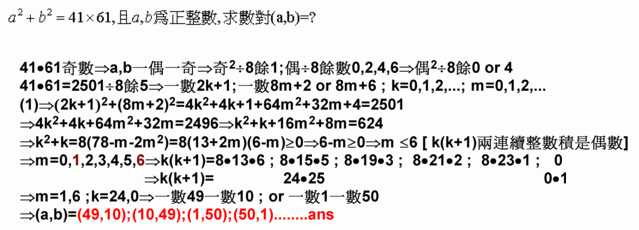## [數學]請教數對解題### [數學]請教數對解題ksjeng### Re: [數學]請教數對解題

ksjeng 寫到:41*61=2501

(1, 50)
24歲不吸菸 沒錢沒馬子colanpaab=(k^2-2501)/2
a,b為
X^2-kX+(k^2-2501)/2=0兩根

so  2501<k^2<5002
ab=(k^2-2501)/2必須為正整數

(1,50)
(10,49)

essential isolated singularity### [數學]我懂了,但懇請進一步賜教

D=5002-k^2必須為完全平方數
(因為ab>0,D>0的關係)(剛剛想通)
so  2501<k^2<5002
k為奇數(卡住了,懇請進一步賜教,奇偶數的判斷這部份我很弱)

(1,50)
(10,49)

ksjeng### [數學]另一種解法

a^2 + b^2 = 41*61

41*61 is an odd number ==> one of a and b must be and odd number and the other must be even.
observe that if (x,y) is a solution, (y,x) is also a solution.
Without loss of generality, we can assume a is odd and b is even
then we can write a=2n+1 and b=2m, for some non negative integer n and positive integer m.

(2n+1)^2 + (2m)^2 = 2501
4n^2 + 4n + 4m^2 = 2500
n^2 + n + m^2 = 625

n^2 + n is always even and 625 is odd ==> m is odd
let m = 2k+1 for some non negative integer k

n^2 + n + (2k+1)^2 = 625
n^2 + n + 4k^2 + 4k = 624

4k^2 + 4k and 624 are divisible by 8
==> n^2 + n is divisible by 8
==> n = 0 or -1 ( mod 8 )

if n = 0 ( mod 8 )
let n = 8h for some non negative integer h
(8h)^2 + 8h + 4k^2 + 4k = 624
16h^2 + 2h + k^2 + k = 156
Since k is non negative, the possible values of h are 0, 1, 2, 3
( otherwise, 16h^2 + 2h + k^2 + k > 156)
substitute the values of h into the equation and solve for k
the only integer solutions of (h,k) are: (0,12) or (3,2)
==> (a,b) = (1,50) or (49,10)
from the observation above, (a,b) can also be (50,1) or (10,49)

if n = -1 ( mod 8 )
let n = 8h-1
(8h-1)^2 + 8h-1 + 4k^2 + 4k = 624
16h^2 - 2h + k^2 + k = 156
Again, since k is non negative, the possible values of h are 0, 1, 2, 3
( otherwise, 16h^2 - 2h + k^2 + k > 156)
substitute the values of h into the equation and solve for k
the only integer solution of (h,k) is: (0,12) (again......)

so, the only solutions of (a,b) are (1,50) or (49,10) or (50,1) or (10,49)

ksjeng### Re: [測試]歪樓樓

ksjengksjeng### [數學]yes### Re: [數學]

ksjeng